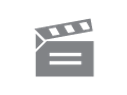Media not available in the Digital Archive
Description
The programme looks at several types of vectors. displacement, velocity, force, and rotation vectors, in a number of practical situations.
Module code and title: MS283, An introduction to calculus MS283; 13 15-08-1979 1979 00:23:52 + Show more... Ted Smith Alan Durrant; Anne Walton BBC Open University Aeroplane routes; Ducks crossing; Yacht masts Alan Durrant introduces the programme with an example of displacement vectors. He uses a map of the UK to illustrate a journey between cities which can be modelled by displacement vectors. Durrant introduces the triangle rule for vectors at the same time. Anne Walton conducts an experiment to test the triangle rule. A model of a flowing river and some mechanical ducks crossing the river demonstrate that velocity vectors combine by the triangle rule. Alan Durrant conducts an experiment to test the triangle rule on force vectors. He uses a physical model made up of string and weights. Another experiment is conducted by Anne Walton to test the triangle rule on rotating objects. Various geometric vectors are induced by varying the direction of rotation and predictions are made. AlIan Durrant sums up the programme so far and introduces a situation where vector calculation can be used in a practical way; in this case the calculation of forces acting on the mast of a sailing dinghy. Anne Walton and Alan Durrant demonstrate how vector forces can be calculated numerically rather than geometrically. They calculate the vector forces acting on the mast of the sailing dinghy above. 6HT/73009 00525_4306 458 no